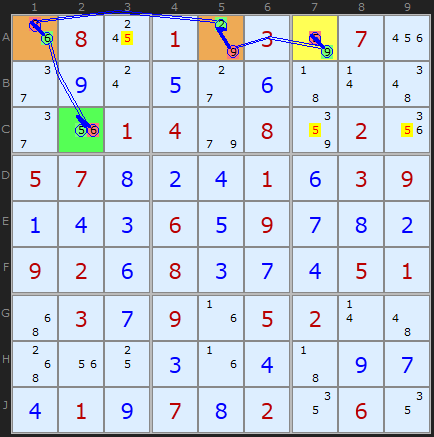Main Page - Back

# XY-Chains

From sudokuwiki.org, the puzzle solver's siteXY-Chain example 1 : Load Example or : From the Start The Y-Wing Chains are infact part of a more encompassing strategy called XY-Chains. The commonality is the same pincer-like attack on candidates that both ends can see and that the chain is made of bi-value cells. With Y-Chains the hinge was expanded to a chain of identical bi-value cells but in an XY-Chain these can be different - as long as there is one candidate to make all the links. The "X" and the "Y" in the name represent these two values in each chain link.

The example here is a very simple XY-Chain of length 4 which removed all 5's highlighted in yellow. The chain ends are 5 A7 and C2 - so all cells that can see both of these are under fire. It's possible to start at either end but lets follow the example from A7. We can reason as follows

• If A7 is 5 then A3/C7/C9 cannot be.
• if A7 is NOT 5 then it's 9, so A5 must be 2, which forces A1 to be 6. If A1 is 6 then C2 is 5.

Which ever choice in A7 the 5's in A3/C7/C9 cannot be 5. The same logic can be traced from C2 to A7 so the strategy is bi-directional, in the jargon.